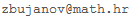Zvonimir Bujanović
Associate Professor at Department of Mathematics
University of Zagreb
Bijenička cesta 30
10000 Zagreb
Croatia

e-mail:office: A307/III
phone: ++385 1 460-5898

## Research interests

• Randomized algorithms in numerical linear algebra
• Numerical solution of large-scale eigenvalue problems
• Matrix theory (perturbation theory, spectral problems)
• Jacobi methods for eigenvalue and SVD problems
• High performance computing (GPU, multicore)
• Numerical solution of matrix equations